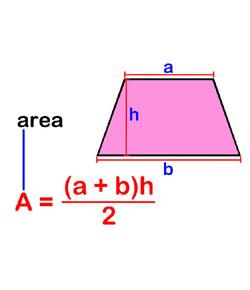# Perimeter Area

9 Questions | Total Attempts: 905SettingsRelated Topics
• 1.
Find the Perimeter and Area of each figure.
• A.

P= 11m A= 28 squared meters

• B.

P= 28m A= 784 squared meters

• C.

P= 28m A= 11 squared meters

• D.

P= 22m A= 28 squared meters

• 2.
Find the perimeter and area of the figure
• A.

P= 22in A= 28 Square inches

• B.

P= 28in A= 48 Square inches

• C.

P= 14 in A= 576 Square inches

• D.

P= 21in A= 48 Square inches

• 3.
Find the perimeter and area of the figure
• A.

P=16 yds A= 60 square yards

• B.

P=16 yds A= 3,600 square yards

• C.

P=32 yds A= 16 square yards

• D.

P=32 yds A= 60 square yards

• 4.
Find the perimeter and area of the figure
• A.

P= 25 ft A= 81 square feet

• B.

P= 36 ft A= 81 square feet

• C.

P= 25 ft A= 6,561 square feet

• D.

P= 36 ft A= 18 square feet

• 5.
Find the perimeter and area of the figure
• A.

P= 16 yds A= 35 square yards

• B.

P= 25yds A= 81 yards

• C.

P= 24 yds A= 35 square yards

• D.

P= 25 yds A= 1,225 square yards

• 6.
Find the Area of the Triangle.
• A.

A= 30 squared m

• B.

A= 15 squared m

• C.

A= 60 squared m

• D.

A = 11 squared m

• 7.
• A.

There are 3 regular(equilateral) Triangles

• B.

There are 2 regular(equilateral) Triangles

• C.

There are 4 regular(equilateral) Triangles

• D.

There are 5 regular(equilateral) Triangles

• 8.
• A.

A = 12 squared cm

• B.

A = 60 cm

• C.

A = 17 squared centimeters

• D.

A = 60 squared cm

• 9.
Is [your statement here] true or false?
• A.

True

• B.

False